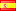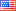# What’s new in PHP 7.3?The PHP 7.3 was released on December 2018, although were not much improvements as it were in the version 7 and version 7.1 it is worth to check the news added features.

If your GNU/Linux distribution does not have the 7.3 version in its official repositories, see How to compile PHP-7.3 en Debian

## 1. Flexible Heredoc and Nowdoc Syntaxes

The closing marker no longer needs to be followed by a semicolon or a new line. In addition, the closing marker may be indented, in which case all lines must have the same or greater indentation than the closing marker.

I think that indenting the closing marker is a good improvement for code readability since before version 7.3 using Heredoc or Nowdoc meant breaking the indentation of the code, let’s see an example.

### Before PHP 7.3

``````<?php
\$sql = <<<SQL
SELECT col1, col2, col3
FROM table1 t1
INNER JOIN table2 t2 ON t2.table1_id = t1.id
SQL;
?>
``````

### As PHP 7.3

``````<?php
\$sql = <<<SQL
SELECT col1, col2, col3
FROM table1 t1
INNER JOIN table2 t2 ON t2.table1_id = t1.id
SQL;
?>
``````

## 2. Array Destructuring supports Reference Assignments

It is now possible to do assignments by reference (using the syntax [&\$a, [\$b, &\$c]] = \$d) when destructuring an array, let’s look at the following example:

``````<?php
<?php
\$countries = [
['SPAIN',   ['EUR', 'ES']],
['USA',     ['USD', 'EN']],
['CUBA',    ['CUC', 'CU']],
['FRANCE',  ['EUR', 'FR']]
];

foreach (\$countries as [&\$country_name, [&\$currency, \$code]]) {
if (\$code == 'CU') {
\$country_name = 'Cuba';
\$currency = 'CUP';
}
}

var_dump(\$countries);

// Print
// array (size=5)
//   0 =>
//     array (size=2)
//       0 => string 'SPAIN' (length=5)
//       1 =>
//         array (size=2)
//           0 => string 'EUR' (length=3)
//           1 => string 'ES' (length=2)
//   1 =>
//     array (size=2)
//       0 => string 'USA' (length=3)
//       1 =>
//         array (size=2)
//           0 => string 'USD' (length=3)
//           1 => string 'EN' (length=2)
//   2 =>
//     array (size=2)
//       0 => string 'ECUADOR' (length=7)
//       1 =>
//         array (size=2)
//           0 => string 'USD' (length=3)
//           1 => string 'EC' (length=2)
//   3 =>
//     array (size=2)
//       0 => string 'Cuba' (length=4)
//       1 =>
//         array (size=2)
//           0 => string 'CUP' (length=3)
//           1 => string 'CU' (length=2)
//   4 =>
//     array (size=2)
//       0 => string 'FRANCE' (length=6)
//       1 =>
//         array (size=2)
//           0 => string 'EUR' (length=3)
//           1 => string 'FR' (length=2)
``````

If we try to execute the previous code in a version prior to version 7.3 PHP throws the following error:

``````Fatal error: [] and list() assignments cannot be by reference
``````

## 3. Instanceof Operator accepts Literals

instanceof now allows literals as the first operand, in which case the result is always FALSE.

``````<?php
// Imprime falso
var_dump(4 instanceof stdClass);
``````

Before PHP-7.3 the following error is thrown:

``````Fatal error: instanceof expects an object instance, constant given
``````

I don’t really know why the developers added this feature because I don’t see a practical use for it.

## 4. CompileError Exception instead of some Compilation Errors

PHP keeps moving from its traditional error handling system to a hierarchy of exceptions: a new CompileError exception has been added, from which ParseError inherits. A small number of compilation errors will now throw a CompileError instead of generating a fatal error.

Note that this does not mean that the PHP engine will throw exceptions when it find a syntax error in our PHP files else refers to the fact of compiling/evaluating PHP code from our source code for example using the function `eval`.

``````<?php
try {
\$val = eval('<?php echo ?>');
} catch (ParseError \$e) {
echo \$e->getMessage();
// Print
// syntax error, unexpected '<', expecting end of file
}
``````

## 5. Trailing Commas are allowed in Calls

Method and function calls allow trailing commas in call parameters.

``````<?php
function my(\$p1, \$p2) {
echo "\$p1 \$p2";
}
// Imprime Hola Mundo!
my('Hola', 'Mundo!',);
``````

Before PHP 7.3 the above code thrown the following error:

``````Parse error: syntax error, unexpected ')'
``````

## 6. Argon2id Support

Argon2 is a password-hashing function created by by Alex Biryukov, Daniel Dinu, and Dmitry Khovratovich and winning of the Password Hashing Competition (PHC). Argon2 is in the public domain and can be downloaded from GitHub.

The –with-password-argon2[=dir] configure argument now provides support for both Argon2i and Argon2id hashes in the `password_hash()`, `password_verify()`, `password_get_info()`, and `password_needs_rehash()` functions. Passwords may be hashed and verified using the PASSWORD_ARGON2ID constant. Support for both Argon2i and Argon2id in the password_*() functions now requires PHP be linked against libargon2 reference library ≥ 20161029.

## 7. New functions have been added to the PHP core

• array_key_first(): Gets the first key of an array.

• array_key_last(): Gets the last key of an array.

• gc_status(): Gets information about the garbage collector

``````array (size=4)
'runs' => int 0
'collected' => int 0
'threshold' => int 10001
'roots' => int 1
``````
• hrtime(): Get the system’s high resolution time.

• is_countable(): Verify that the contents of a variable is an array or an object implementing Countable

• net_get_interfaces(): Gets an array of key => value with the information about physical network interfaces.

``````Array
(
[lo] => Array
(
[unicast] => Array
(
 => Array
(
[flags] => 65609
[family] => 17
)

 => Array
(
[flags] => 65609
[family] => 2
)

)

[up] => 1
)

[eth0] => Array
(
[unicast] => Array
(
 => Array
(
[flags] => 69699
[family] => 17
)

 => Array
(
[flags] => 69699
[family] => 2
)

)

[up] => 1
)
)
``````

Check New Functions for all new added functions.### PHP new features, 3 (8)

This site uses Akismet to reduce spam. Learn how your comment data is processed.